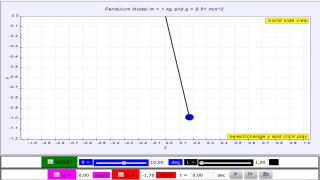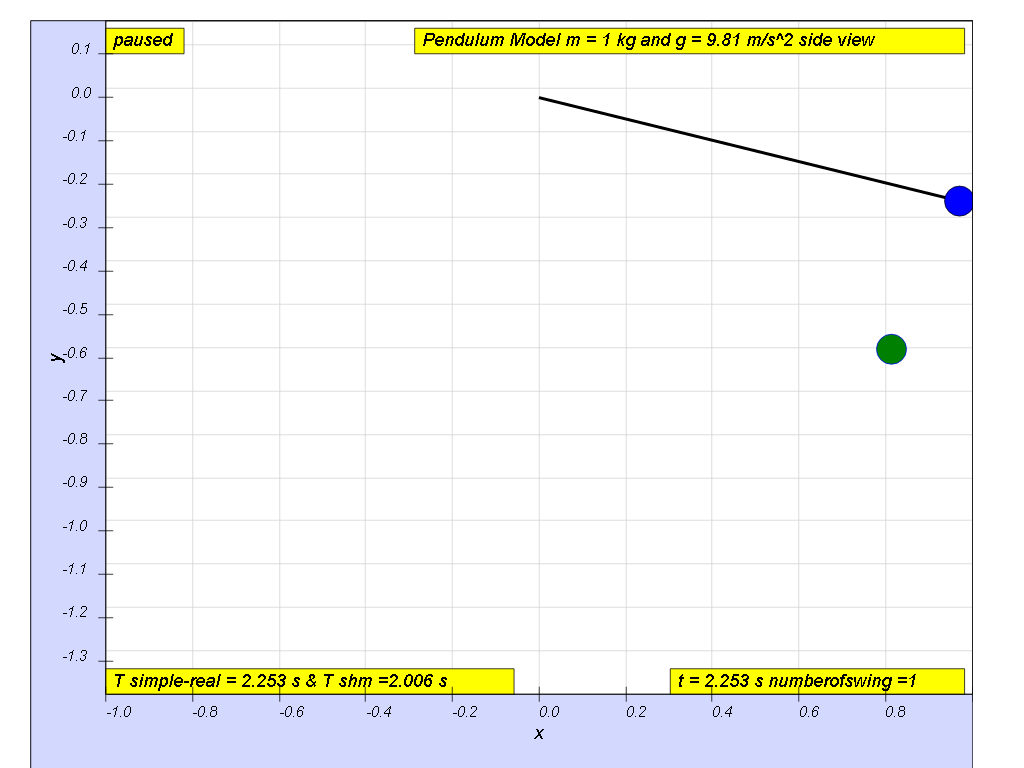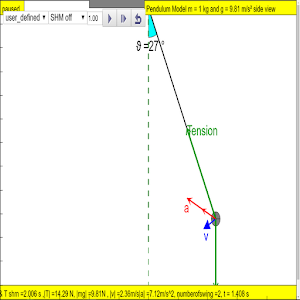## 10.5 Example of investigation of motion of an oscillator using experimental and graphical method HTML5 Applet Simulation ModelChapter SHM Example 01_02

### 1.5 Example of investigate the motion of an oscillator using experimental and graphical methods### 1.5.1.Q1: what is the maximum angle of release before the motion is not accurately described as a simple harmonic motion for the case of a simple free pendulum?

Example 1: Simple pendulum A pendulum bob given an initial horizontal displacement and released to swing freely to produce to and fro motion

### 1.5.2 Suggested Inquiry Steps:

1.     Define the question in your own words
2.     Plan an investigation to explore angle of release to record the actual period T and theoretical period  ${T}_{the\phantom{\rule{1ex}{0ex}}\text{or}\phantom{\rule{1ex}{0ex}}y}=2\pi \sqrt{\frac{L}{g}}$  where L is the length of the mass pendulum of mass, m and g is the gravitational acceleration of which the mass is experiencing, on Earth's surface  g = 9.81 m/s2
3.     A suggested record of the results could look like this
 angle / degree T /s Ttheory / s $err\phantom{\rule{1ex}{0ex}}\text{or}\phantom{\rule{1ex}{0ex}}=\frac{\left(T-{T}_{the\phantom{\rule{1ex}{0ex}}\text{or}\phantom{\rule{1ex}{0ex}}y}\right)}{T}100%$ 05 10 15 20 30 40 50 60 70 80 90

With the evidences collected or otherwise, suggests what the conditions of which the angle of oscillation can the actual period T be approximated to theoretical period such that  T  ≈ ${T}_{the\phantom{\rule{1ex}{0ex}}\text{or}\phantom{\rule{1ex}{0ex}}y}=2\pi \sqrt{\frac{L}{g}}$

angle θ  ≈ 10 degrees for $err\phantom{\rule{1ex}{0ex}}\text{or}\phantom{\rule{1ex}{0ex}}=\frac{\left(2.010-2.006\right)}{2.010}\left(100\right)=0.2%$, depending on what is the error acceptable, small angle is typically about less than 10 degree of swing from the vertical.

### 1.5.4 Conclusion:

Motion approximates simple harmonic motion when the angle of oscillation is small < 10 degrees..

### 1.5.5 Other Interesting fact(s):

Motion approximates SHM when the spring does not exceed limit of proportionality during oscillations.

### 1.5.6 Real Life Application of Small Angle Approximations:

Astronomical applications of the Small Angle Approximation

http://youtu.be/BRbCW2MsL94?t=2m16s This video shows many pendulums that goes in phase and out of phase with each other pendulum to creating a visually stunning effect.

### Translations

Code Language Translator Run### Software Requirements

SoftwareRequirements

 Android iOS Windows MacOS with best with Chrome Chrome Chrome Chrome support full-screen? Yes. Chrome/Opera No. Firefox/ Samsung Internet Not yet Yes Yes cannot work on some mobile browser that don't understand JavaScript such as..... cannot work on Internet Explorer 9 and below

### CreditsThis email address is being protected from spambots. You need JavaScript enabled to view it.

### end faq

http://iwant2study.org/lookangejss/02_newtonianmechanics_8oscillations/ejss_model_SHM195/SHM195_Simulation.xhtml

## Appshttps://play.google.com/store/apps/details?id=com.ionicframework.shm195app415781&hl=en